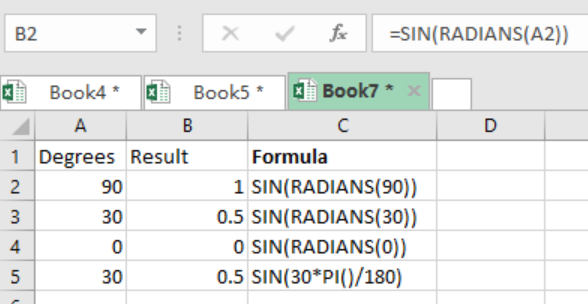Get instant live expert help with Excel or Google Sheets“My Excelchat expert helped me in less than 20 minutes, saving me what would have been 5 hours of work!”

#### Post your problem and you’ll get expert help in seconds.

Your message must be at least 40 characters
Our professional experts are available now. Your privacy is guaranteed.

# An Overview of the Excel SIN Function

The Excel SIN function is fundamental when we want to find the sine of an angle in radians. We need to multiply the angle given by PI()/180 so that we can get it in degrees. We can also use the RADIANS function to convert it into radians. This article provides an overview of the Excel SIN function.Figure 1: Overview of Excel SIN function

## General syntax of the formula

`=SIN(number)`

Where;

• Number- refers to the angle for which we want to find the sine. It has to be in radians.

## Understanding the formula

• For us to use the Excel SIN function, we first need to convert the angles given to radians.
• Supplying the angles to radians can be done by either multiplying the angle by PI()/180 or using the excel RADIANS function.
• In our example in figure 1 above, we have used the RADIANS function to get the angle in radians.
• Below is another example of how you can use PI()/180 to get the same result;
`=SIN(30*PI()/180)`
• This is as shown in row 5 in figure 1 above.

## Instant Connection to an Expert through our Excelchat Service

Most of the time, the problem you will need to solve will be more complex than a simple application of a formula or function. If you want to save hours of research and frustration, try our live Excelchat service! Our Excel Experts are available 24/7 to answer any Excel question you may have. We guarantee a connection within 30 seconds and a customized solution within 20 minutes.

Solution examplesIf I merged a multi-row, multi-column selection. Iand types some text into the merged cell and then unmerges it, what will happen to the text?
Solved by S. Y. in 60 minsIF SUM OF RANGE IS MORE THAN ZERO,THEN SUM IF THE RANGE IS BLANK THEN BLANK IF THE RANGE HAS ONLY ZERO,THEN ZERO HOW SHALL I COMBINE THE ABOVE THREE IN A SINGLE CELL??
Solved by M. D. in 18 minsi need a formula to calculate previous day intraday high from google finance to google sheet. I AM USING THIS ONE =GOOGLEFINANCE(A2,"high",today()-1) BUT ITS NOT WORKING AND GIVING ME ERROR "When evaluating GOOGLEFINANCE, the query for the symbol: 'HDFCBANK' returned no data." HERE A2 IS "HDFCBANK" A TICKER.. AND I ALSO DONT WANT DATE COLUMN WITH THE PREVIOUS DAY INTRADAY HIGH PRICE.
Solved by G. E. in 40 mins